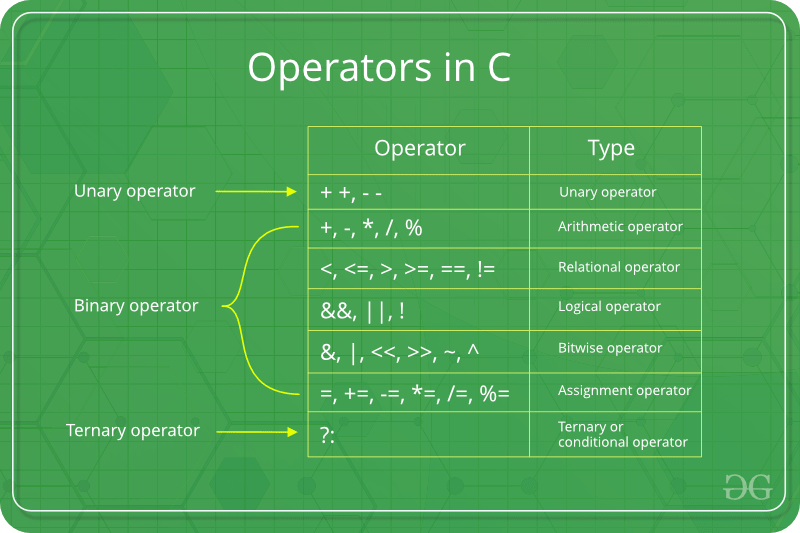# Assignment Operators in C/C++

• Difficulty Level : Medium
• Last Updated : 11 Oct, 2019Assignment operators are used to assigning value to a variable. The left side operand of the assignment operator is a variable and right side operand of the assignment operator is a value. The value on the right side must be of the same data-type of the variable on the left side otherwise the compiler will raise an error.
Different types of assignment operators are shown below:

• “=”: This is the simplest assignment operator. This operator is used to assign the value on the right to the variable on the left.
For example:

```a = 10;
b = 20;
ch = 'y';
```
• “+=”: This operator is combination of ‘+’ and ‘=’ operators. This operator first adds the current value of the variable on left to the value on the right and then assigns the result to the variable on the left.
Example:

```(a += b) can be written as (a = a + b)
```

If initially value stored in a is 5. Then (a += 6) = 11.

• “-=”This operator is combination of ‘-‘ and ‘=’ operators. This operator first subtracts the current value of the variable on left from the value on the right and then assigns the result to the variable on the left.
Example:

```(a -= b) can be written as (a = a - b)
```

If initially value stored in a is 8. Then (a -= 6) = 2.

• “*=”This operator is combination of ‘*’ and ‘=’ operators. This operator first multiplies the current value of the variable on left to the value on the right and then assigns the result to the variable on the left.
Example:

```(a *= b) can be written as (a = a * b)
```

If initially value stored in a is 5. Then (a *= 6) = 30.

• “/=”This operator is combination of ‘/’ and ‘=’ operators. This operator first divides the current value of the variable on left by the value on the right and then assigns the result to the variable on the left.
Example:

```(a /= b) can be written as (a = a / b)
```

If initially value stored in a is 6. Then (a /= 2) = 3.

Below example illustrates the various Assignment Operators:

## C

 `// C program to demonstrate ` `// working of Assignment operators ` ` `  `#include ` ` `  `int` `main() ` `{ ` ` `  `    ``// Assigning value 10 to a ` `    ``// using "=" operator ` `    ``int` `a = 10; ` `    ``printf``(``"Value of a is %d\n"``, a); ` ` `  `    ``// Assigning value by adding 10 to a ` `    ``// using "+=" operator ` `    ``a += 10; ` `    ``printf``(``"Value of a is %d\n"``, a); ` ` `  `    ``// Assigning value by subtracting 10 from a ` `    ``// using "-=" operator ` `    ``a -= 10; ` `    ``printf``(``"Value of a is %d\n"``, a); ` ` `  `    ``// Assigning value by multiplying 10 to a ` `    ``// using "*=" operator ` `    ``a *= 10; ` `    ``printf``(``"Value of a is %d\n"``, a); ` ` `  `    ``// Assigning value by dividing 10 from a ` `    ``// using "/=" operator ` `    ``a /= 10; ` `    ``printf``(``"Value of a is %d\n"``, a); ` ` `  `    ``return` `0; ` `} `

## C++

 `// C++ program to demonstrate  ` `// working of Assignment operators  ` ` `  `#include ` `using` `namespace` `std; ` ` `  `int` `main()  ` `{  ` ` `  `    ``// Assigning value 10 to a  ` `    ``// using "=" operator  ` `    ``int` `a = 10;  ` `    ``cout << ``"Value of a is "``<

Output:

```Value of a is 10
Value of a is 20
Value of a is 10
Value of a is 100
Value of a is 10
```

My Personal Notes arrow_drop_up
Recommended Articles
Page :